# How to list all dates between two dates in Excel?

Imagine you are in a scenario where you need to construct a table that is organized by date. For instance, you are making a schedule and need to specify a list of activities arranged by date in the appropriate order. You would require a list of dates in the event that this occurs.

There is no such thing as a limitless list, so it stands to reason that the one you would have a beginning and an end date. In certain circumstances, if you already know the date at which you want to begin and the date at which you want to finish, you may need to use Excel to create a list of all the dates that fall in between these two specified days.

## List all Dates using Formula

Let’s understand step by step through an example.

Step 1

In our example, we have Start Date and End Date in an Excel sheet. We will create a list the dates in between the starting date and ending date. See the following image.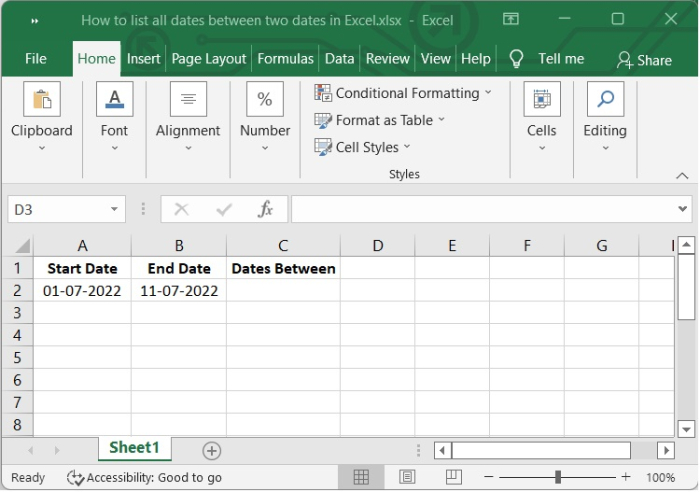Step 2

Then type the below given formula in C2 cell and press enter. The formula is =A2+1 where A2 is the cell in which starting date is written. After pressing Enter, you will see the next date of starting date. See the following image.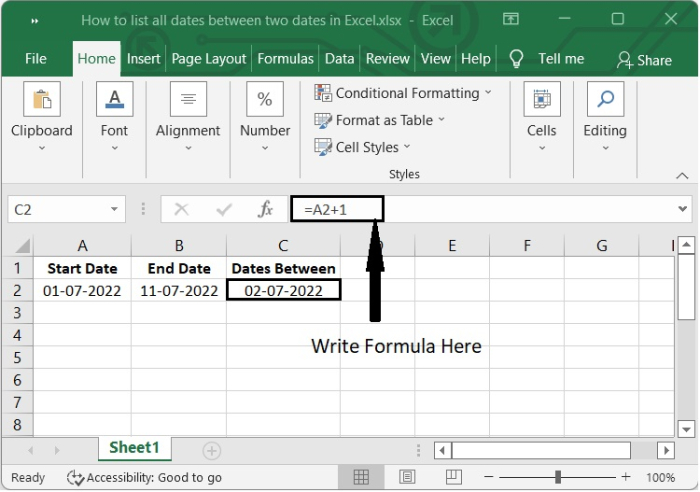Step 3

After that, select the cell C3 and type the below given formula in formula bar and press enter.

=IF($A$2+ROW(A2)>=$B$2,"",C2+1)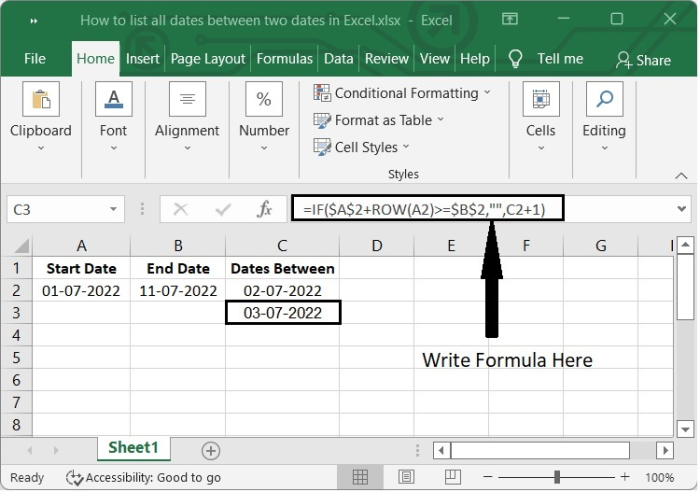Step 4

Click the "+" sign appears on the lower right corner of the cell C3, which activates the autofill function and then drag down to reflect in other cells.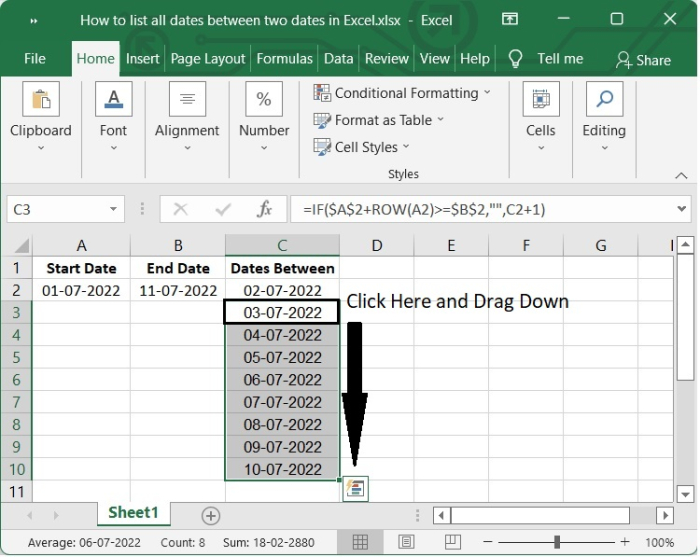The difficulty in defining the syntax or making modifications to this formula is the primary drawback to using it. In addition to this, the dates aren't quite in the correct order.

## List All Dates Using VBA

If you are interested in macro code, the following Visual Basic for Applications (VBA) can be used in Excel to list any dates that fall between two specified dates.

Follow the steps given below −

Step 1

Enter the Start date and the End date into separate cells.Step 2

To open the Microsoft Visual Basic for Applications window, press the "Alt + F11" keys simultaneously.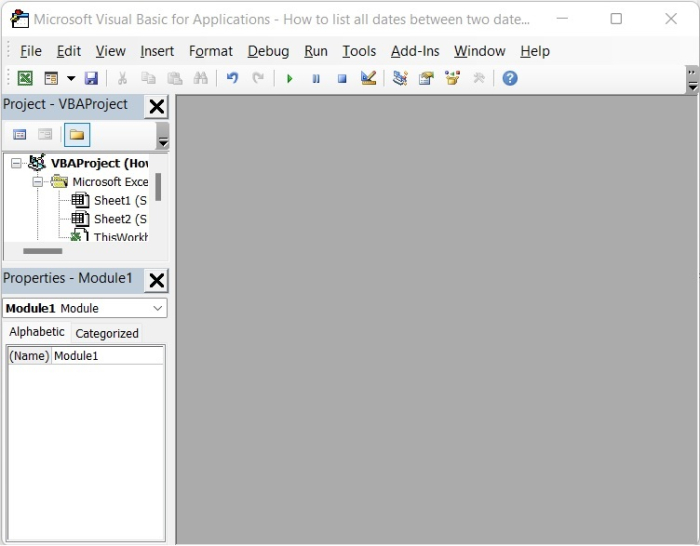Step 3

Then click Insert > Module and the popup Module window will open.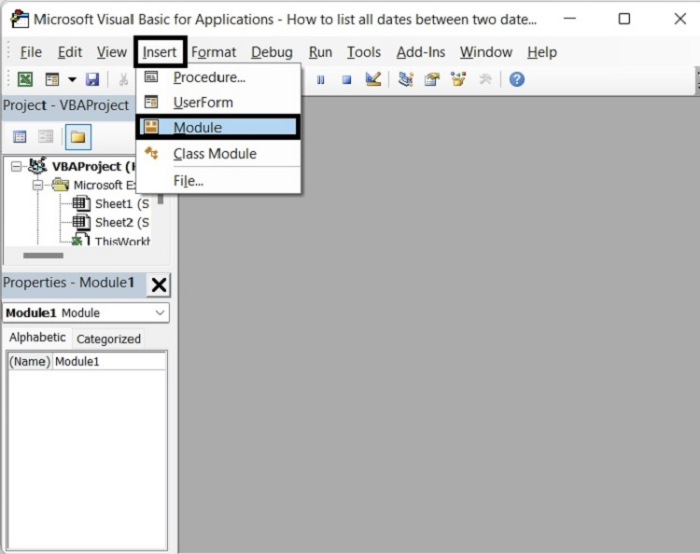This is the VBA editor.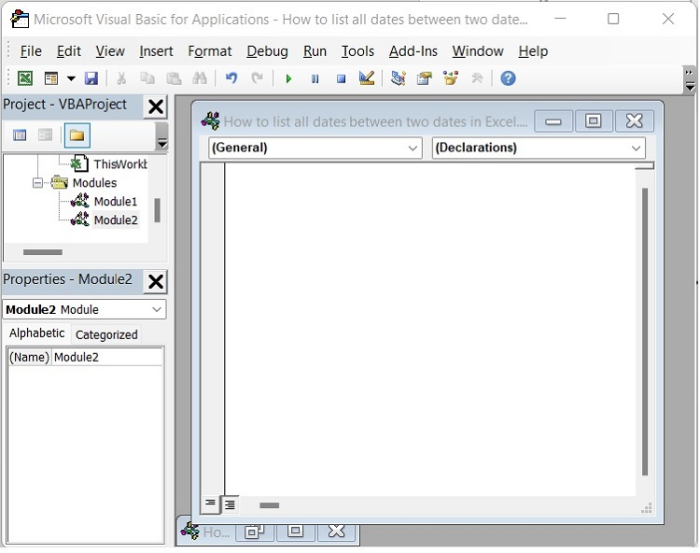Step 4

In the Module window, type the following VBA code.

Sub DatesBetween()
Dim rng As Range
Dim StartRng As Range
Dim EndRng As Range
Dim OutRng As Range
Dim StartValue As Variant
Dim EndValue As Variant
xTitleId = "VBOutput"
Set StartRng = Application.Selection
Set StartRng = Application.InputBox("Start Range (single cell):", xTitleId, StartRng.Address, Type:=8)
Set EndRng = Application.InputBox("End Range (single cell):", xTitleId, Type:=8)
Set OutRng = Application.InputBox("Out put to (single cell):", xTitleId, Type:=8)
Set OutRng = OutRng.Range("A1")
StartValue = StartRng.Range("A2").Value
EndValue = EndRng.Range("A2").Value
If EndValue - StartValue <= 0 Then
Exit Sub
End If
ColIndex = 0
For i = StartValue To EndValue
OutRng.Offset(ColIndex, 0) = i
ColIndex = ColIndex + 1
Next
End Sub

Step 5

Click Run or F5 to run the VBA, a window pops up where you will choose the starting date, then click OK, then in the next window, choose the Ending date, then click OK.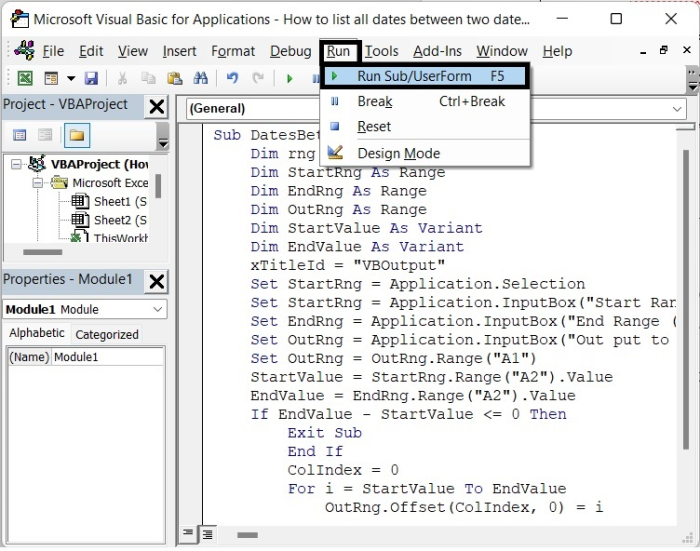Then another window pops up asking Output to, give the output cell and click OK.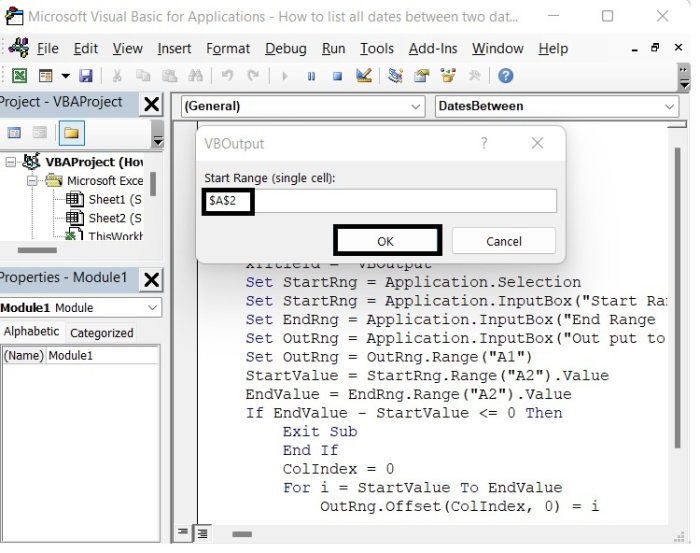In our example, the Cell value of Starting Date is A2, so we write it in this way: "$A$$A$2".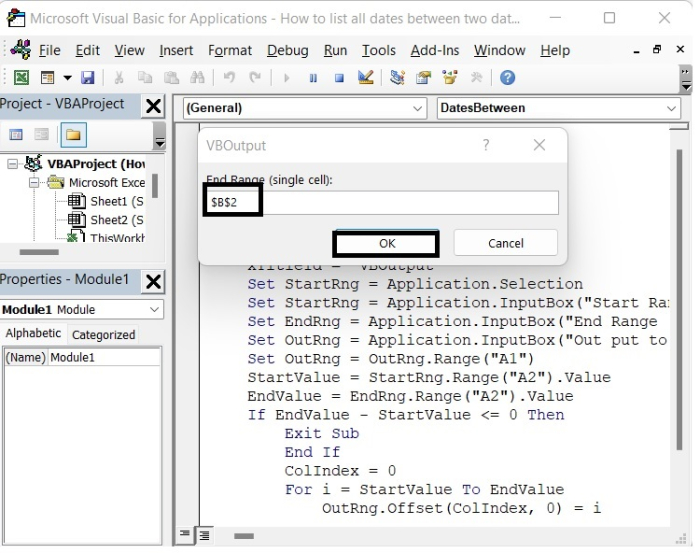In our example, the Cell value of Ending Date is B2, so we write it in this way: "$B$$B$2".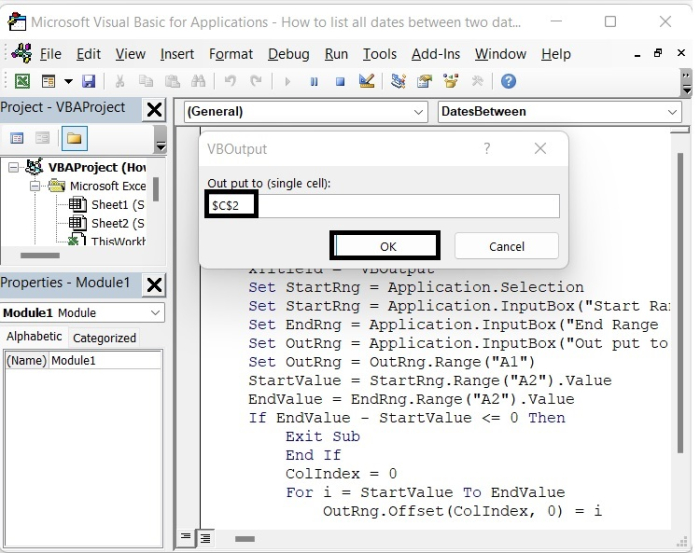In our example, the Cell value of Output Date is C2, so we write it in this way: "$C$$C$2".

After clicking OK, close the VBA window and you will get the dates between the starting and ending dates, including both starting and ending dates, as shown in the following image.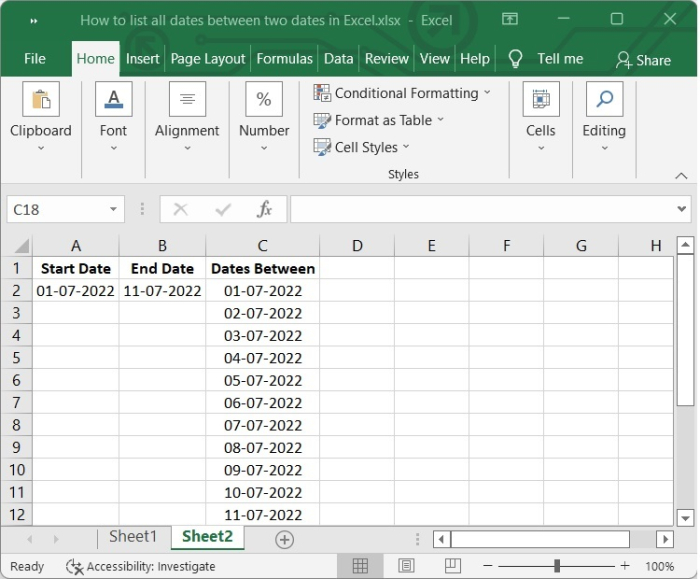## Conclusion

In this tutorial, we showed two different ways (Formula and VBA) that you can use to list all the dates between two given dates in Excel.